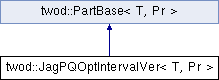# twod::JagPQOptIntervalVer< T, Pr > Class Template Reference

optimal PxQ jagged algorithm from "Ali Pinar and Cevdet Aykanat, Sparse Matrix Decomposition with Optimal Load Balancing, HiPC 1997" using the second dimension as the main dimension. More...

`#include <twod/jag_pq_opt_interval.hpp>`

Inheritance diagram for twod::JagPQOptIntervalVer< T, Pr >:## Detailed Description

### template<typename T, typename Pr> class twod::JagPQOptIntervalVer< T, Pr >

optimal PxQ jagged algorithm from "Ali Pinar and Cevdet Aykanat, Sparse Matrix Decomposition with Optimal Load Balancing, HiPC 1997" using the second dimension as the main dimension.

This class is implemented as a wrapper to twod::JagPQOptIntervalHor.

Parameters:
 T type of the data in the prefix sum array Pr type of the prefixsum array

List of all members.

## Public Member Functions

JagPQOptIntervalVer ()
void setP (int P)
selects the number of stripes in the main dimension
virtual T part (int procCount, const Pr &prefixSumArray, util::RectList< T, Pr > &parts)
Applies a 2d partitioning algorithm to a given 2d prefixSumArray .
virtual ~JagPQOptIntervalVer ()

int P

## Constructor & Destructor Documentation

template<typename T , typename Pr >
 twod::JagPQOptIntervalVer< T, Pr >::JagPQOptIntervalVer ( )
template<typename T , typename Pr >
 twod::JagPQOptIntervalVer< T, Pr >::~JagPQOptIntervalVer ( ) ` [virtual]`

## Member Function Documentation

template<typename T , typename Pr >
 T twod::JagPQOptIntervalVer< T, Pr >::part ( int procCount, const Pr & prefixSumArray, util::RectList< T, Pr > & parts ) ` [virtual]`

Applies a 2d partitioning algorithm to a given 2d prefixSumArray .

Parameters:
 [in] procCount is the number of processors [in] prefixSumArray first column and first row consists of zero only. But the borders of rectangles in rect_list never touch this area (index 0 in row or column) [out] parts must be empty before calling this function. It will contain the partition after the function return
Returns:

Implements twod::PartBase< T, Pr >.

template<typename T , typename Pr >
 void twod::JagPQOptIntervalVer< T, Pr >::setP ( int P )

selects the number of stripes in the main dimension

Parameters:
 P number of stripes in the main dimension. If 0, the number of stripes will be computed at runtime by taking the square root of the number of processors.

## Member Data Documentation

template<typename T, typename Pr>
 int twod::JagPQOptIntervalVer< T, Pr >::P` [private]`

The documentation for this class was generated from the following files: